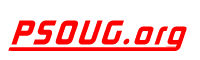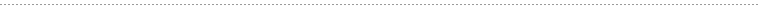Quick Search:Snippet Name: Accumulate from different sources

Description: This code accumulates 100 units of part number 9999 from various storage bins

Comment: (none)

Language: PL/SQL
Highlight Mode: PLSQL

```/*
** This code accumulates 100 units of part number 9999 from
** various storage bins.
*/
DECLARE
CURSOR bin_cur(part_number NUMBER) IS SELECT amt_in_bin
FROM bins
WHERE part_num = part_number AND
amt_in_bin > 0
ORDER BY bin_num
FOR UPDATE OF amt_in_bin;
bin_amt         bins.amt_in_bin%TYPE;
total_so_far    NUMBER(5) := 0;
amount_needed   CONSTANT NUMBER(5) := 100;
bins_looked_at  NUMBER(3) := 0;
BEGIN
OPEN bin_cur(9999);
WHILE total_so_far < amount_needed LOOP
FETCH bin_cur INTO bin_amt;
EXIT WHEN bin_cur%NOTFOUND;
/* If we exit, there's not enough to *
* satisfy the order.                */
bins_looked_at := bins_looked_at + 1;
IF total_so_far + bin_amt < amount_needed THEN
UPDATE bins SET amt_in_bin = 0
WHERE CURRENT OF bin_cur;
-- take everything in the bin
total_so_far := total_so_far + bin_amt;
ELSE        -- we finally have enough
UPDATE bins SET amt_in_bin = amt_in_bin
- (amount_needed - total_so_far)
WHERE CURRENT OF bin_cur;
total_so_far := amount_needed;
END IF;
END LOOP;
CLOSE bin_cur;
INSERT INTO temp VALUES (NULL, bins_looked_at, '<- bins looked at');
COMMIT;
END;
/
```